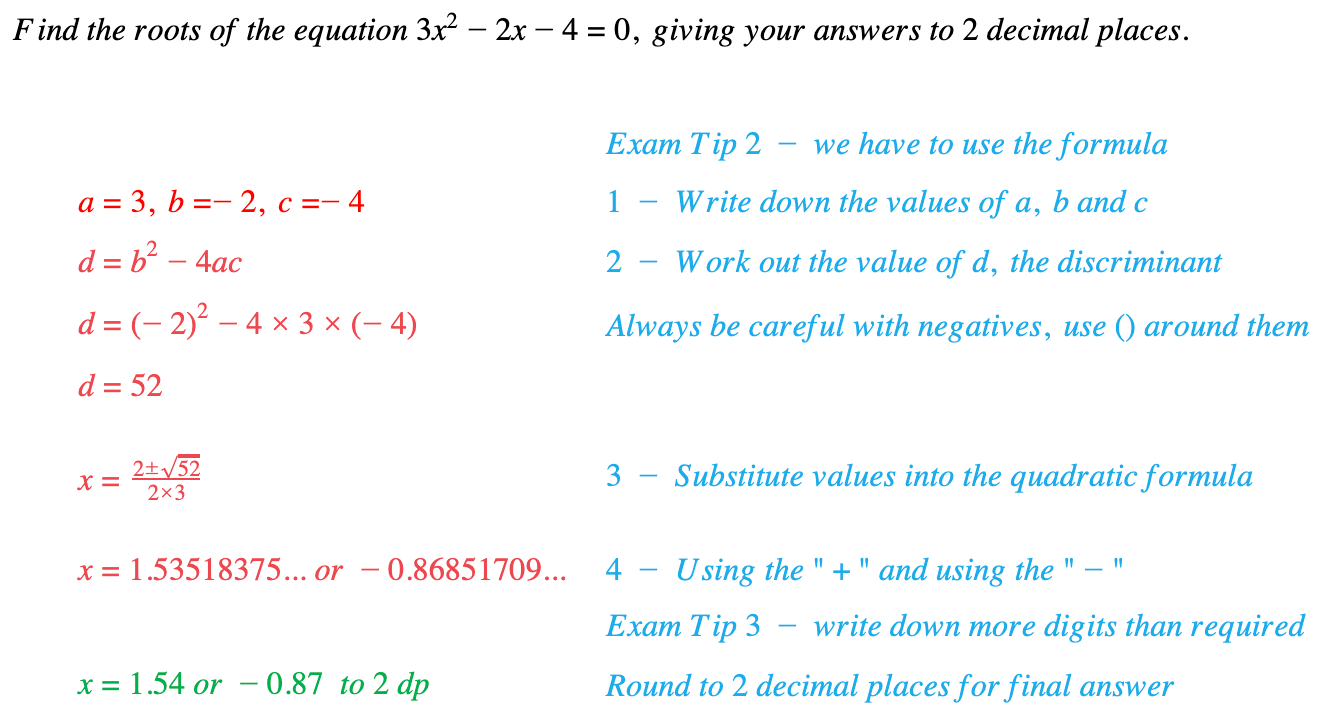# Edexcel IGCSE Maths 复习笔记 2.14.1 Quadratic Formula

Edexcel IGCSE Maths 复习笔记 2.14.1 Quadratic Formula

#### What is a quadratic equation?

• A quadratic equation looks like (or can/should be made to look like) this:ax2 + bx + c = 0 (as long as a ≠ 0)

#### What is the quadratic formula?

• The Quadratic Formula is a way of finding the roots (also called solutions) of a quadratic equation:• The part of the formula under the square root (b2 – 4ac) is called the discriminant and it tells you a lot about the roots:
• If b2 – 4ac > 0 then there are two distinct (different) real roots (*)
• If b2 – 4ac = 0 then there is one real root (two repeated roots)
• If b2 – 4ac < 0 then there are no real roots (and the equation cannot be solved)

• Also (and not a lot of people know this!):
• If b2 – 4ac is a perfect square ( 1, 4, 9, 16, …) then the quadratic can be factorised

(*) We have to call them “real” roots for a very mathsy reason – don’t worry about it too much – all the numbers you come across at GCSE are real!

#### A quick note about calculators

If you have an “advanced” scientific calculator (like ones from the Casio Classwiz range) you may find it will solve quadratic equations for you. However, at GCSE, you should avoid using this feature as marks are awarded for method. At best you can use them to check your final answers but be aware that such calculators often show solutions even when the roots are not real.

#### When do you use the quadratic formula?

You can use the Quadratic Formula whenever you need to solve a quadratic equation UNLESS you are specifically asked to do it by Factorising or Completing the Square. (You could still use the formula to check your answer!)

If the question asks you to give your answers to a certain degree of accuracy (eg 3 significant figures or 2 decimal places etc) then you will not be able to factorise and you MUST use the formula.

#### How do you use the quadratic formula?

• The safest way to avoid errors is to break the formula down into stages:
1. WRITE DOWN the values of a, b and c – ie a = … , b = … and c = …
2. Work out the DISCRIMINANT, d = b2 – 4ac
3. Substitute values into the QUADRATIC FORMULA including d
4. Work out the TWO ANSWERS on your calculator(Type in the formula twice, once with the “+” and once with the “-”)

#### Exam Tip

Write down more digits than you need from your calculator display.

Then do the rounding as a separate stage.

This will avoid errors and could save you a mark.

#### Worked Example